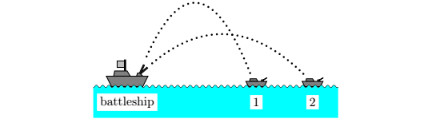# Problem: Given: The battleship and enemy ships 1 and 2 lie along a straight line. Neglect air friction. Consider the motion of the two projectiles fired at t = 0. Their initial speeds are different and they reach different maximum heights h1 and h2. What is the ratio of the time of flight, t 1 and t2 respectively, that the shells reach?1. t1 / t2 = 2 (√ h1 / h2)2. t1 / t2 = 1/ √2 (√ h1 / h2)3. t1 / t2 = 2 (√ h2 / h1)4. t1 / t2 = 1/2 (√ h1 / h2)5. t1 / t2 = (√ h1 / h2)6. t1 / t2 = 1/2 (√ h2 / h1)7. t1 / t2 = h2 / h18. t1 / t2 = √2 (√ h1 / h2)9. t1 / t2 = (√ h2 / h1)10. t1 / t2 = (h1 / h2)

###### FREE Expert Solution
86% (176 ratings)
###### Problem Details

Given: The battleship and enemy ships 1 and 2 lie along a straight line. Neglect air friction. Consider the motion of the two projectiles fired at t = 0. Their initial speeds are different and they reach different maximum heights hand h2. What is the ratio of the time of flight, t 1 and t2 respectively, that the shells reach?

1. t1 / t2 = 2 (√ h1 / h2)

2. t1 / t2 = 1/ √2 (√ h1 / h2)

3. t1 / t2 = 2 (√ h2 / h1)

4. t1 / t2 = 1/2 (√ h1 / h2)

5. t1 / t2 = (√ h1 / h2)

6. t1 / t2 = 1/2 (√ h2 / h1)

7. t1 / t2 = h2 / h1

8. t1 / t2 = √2 (√ h1 / h2)

9. t1 / t2 = (√ h2 / h1)

10. t1 / t2 = (h1 / h2)